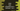# 3 ways to find perfect numbers in a range in Java## How to find perfect numbers in a range in Java:

This post will explain how to write a Java program to find all perfect numbers in a given range. You will understand how to check if a number is a perfect number or not and how to find all perfect numbers in a given range.

### What is a perfect number:

A number is called a perfect number if the number is equal to the sum of its factors, excluding the number itself.

For example, 6 is a perfect number because the sum of its factors is 1 + 2 + 3 which is equal to 6.

So, we need to find the factors of a number and by comparing the sum of the factors with the number itself, we can say if the number is a perfect number or not.

### Example 1: Java program to find the perfect numbers in a given range:

The below program shows how to find all the perfect numbers in a user-given range:

``````import java.util.Scanner;

public class Main {

private static boolean isPerfect(int n) {
int sum = 1;
for (int i = 2; i < n; i++) {
if (n % i == 0)
sum += i;
}
return sum == n && n != 1;
}

public static void main(String[] args) {
Scanner scanner = new Scanner(System.in);
int start, end;

System.out.println("Enter the start of the range: ");
start = scanner.nextInt();

System.out.println("Enter the end of the range: ");
end = scanner.nextInt();

System.out.println("Perfect numbers in between " + start + " and " + end + " are:");
for (int no = start; no <= end; no++) {
if (isPerfect(no)) {
System.out.println(no);
}
}
}
}``````
• It uses a Scanner variable to read the start and end of the range.
• It uses a for loop to find all perfect numbers between start and end(inclusive).
• On each iteration, it calls the isPerfect method to check if the number is a perfect number or not.
• The isPerfect method takes one number as its parameter and returns one boolean value defining if the number is a perfect number or not.
• It initializes one variable sum as 1 to hold the sum of the factors of the number. It is initialized as 1 as 1 is the factor of all numbers.
• It runs one for loop from i = 2 to i = n - 1, where n is the given number.
• On each iteration, it checks if i can divide the number n or not. If yes, it will add that value to the sum variable.
• At the end of this method, it returns true if the calculated sum is equal to the given number and if it is not equal to 1. Else, it returns false.

### Example 2: Java program to find the perfect numbers in a given range by iterating up to half of the number:

We can reduce the iteration by half. Instead of iterating from 2 to number - 1, we can iterate from 2 to number/2 as larger than this value can’t be a factor of the number.

The final program become:

``````import java.util.Scanner;

public class Main {

private static boolean isPerfect(int n) {
int sum = 1;
for (int i = 2; i <= n/2; i++) {
if (n % i == 0)
sum += i;
}
return sum == n && n != 1;
}

public static void main(String[] args) {
Scanner scanner = new Scanner(System.in);
int start, end;

System.out.println("Enter the start of the range: ");
start = scanner.nextInt();

System.out.println("Enter the end of the range: ");
end = scanner.nextInt();

System.out.println("Perfect numbers in between " + start + " and " + end + " are:");
for (int no = start; no <= end; no++) {
if (isPerfect(no)) {
System.out.println(no);
}
}
}
}``````

The only change in this program is:

``````...
...
for (int i = 2; i <= n/2; i++) {
...``````

It will work similarly to the above program but it will be faster.

### Example 3: Recursive Java program to find the perfect numbers in a given range:

Let’s write down the program recursively. The isPerfect method will recursively check if a number is perfect or not.

``````import java.util.Scanner;

public class Main {

private static boolean isPerfect(int n, int i, int sum) {
if(n == 1)
return false;

if (i > n / 2)
return sum == n;

if (n % i == 0)
return isPerfect(n, i + 1, sum + i);
else
return isPerfect(n, i + 1, sum);
}

public static void main(String[] args) {
Scanner scanner = new Scanner(System.in);
int start, end;

System.out.println("Enter the start of the range: ");
start = scanner.nextInt();

System.out.println("Enter the end of the range: ");
end = scanner.nextInt();

System.out.println("Perfect numbers in between " + start + " and " + end + " are:");
for (int no = start; no <= end; no++) {
if (isPerfect(no, 2, 1)) {
System.out.println(no);
}
}
}
}``````
• The isPerfect method is changed to take three parameters. It takes n, i and sum variables as the parameters.
• This method is called with i = 2 and sum = 1 in the main method.
• Once the value of i increases more than number/2, it checks if the value of sum and n are equal or not and returns that value. Else, it checks if the current value of i is a factor of n or not and calls the isPerfect method recursively.

It will give similar output.

``````Enter the start of the range:
1
Enter the end of the range:
10000
Perfect numbers in between 1 and 10000 are:
6
28
496
8128``````